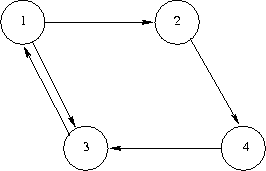2129. Internet graph

The whole of internet can be seen as a huge graph, in which the nodes are the webages and an edge is a hyperlink from one webpage to another webpage. On can start from a webpage and “hop” to several webpages by just clicking on the hyperlinks present in a webpage. Given a graph representing the internet, we want to know what is the average shortest distance between any two webpages.

Note that this graph will be directed. Consider the graph given below. The length of the shortest path from node 1 to nodes 2, 3, and 4 is 1,1, and 2 respectively. From node 2 to nodes 1, 3 and 4, the shortest paths have lengths of 3, 2, and 1. From node 3 to nodes 1, 2, and 4, the shortest paths have lengths of 1, 2, and 3. Finally, from node 4 to nodes 1, 2, and 3 the shortest paths have lengths of 2, 3, and 1. The sum of these path lengths is 1 + 1 + 2 + 3 + 2 + 1 + 1 + 2 + 3 + 2 + 3 + 1 = 22. Since there are 12 possible pairs of nodes to consider, we obtain an average path length of 22/12, or 1.833 (accurate to three fractional digits).输入格式

The input data will have multiple test cases. Each test case will consist of an arbitrary number of pairs of integers, a and b, each representing a link from a page numbered a to a page numbered b. Page numbers will always be in the range 1 to 100. The input for each test case will be terminated with a pair of zeroes, which are not to be treated as page numbers. An additional pair of zeroes will follow the last test case, effectively representing a test case with no links, which is not to be processed. The graph will not include self-referential links (that is, there will be no direct link from a node to itself), and at least one path will exist from each node in the graph to every other node in the graph.

输出格式

For each test case, compute the average shortest path length between every pair of nodes, accurate to 4 places of decimal.

样例

Input
1 2 2 4 1 3 3 1 4 3 0 0
1 2 1 4 4 2 2 7 7 1 0 0
0 0
Output
1.833
1.750

13 人解决，32 人已尝试。

13 份提交通过，共有 48 份提交。

6.7 EMB 奖励。